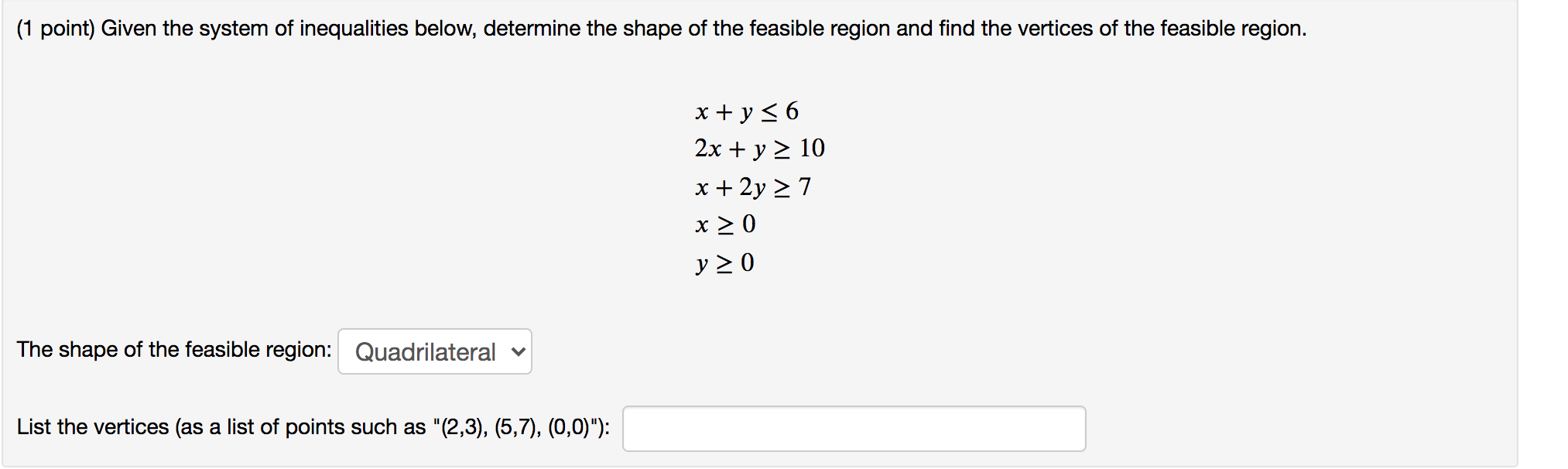# sketch the feasible region for this system of the inqualities and find the coordinates of each vertex:y greater than or equal to 1;x less than or equal to 2; -1x+2y less than or equal to 6;1x+2y greater than or equal to 2

sketch the feasible region for this system of the inqualities and find the coordinates of each vertex:y greater than or equal to 1;x less than or equal to 2; -1x+2y less than or equal to 6;1x+2y greater than or equal to 2

a) y>/= 1
b) x</=2

2y</= x+6
c) y </= (1/2) x + 3

2 y >/= -x +2
d) y >/= -(1/2)x + 1

a and d hit at (0,1)
a and b hit at (2,1)
b and c hit at (2,4)
c and d hit at (-2,3)
Inside that figure
##### Add Answer of: sketch the feasible region for this system of the inqualities and find the coordinates of each vertex:y greater than or equal to 1;x less than or equal to 2; -1x+2y less than or equal to 6;1x+2y greater than or equal to 2
Similar Homework Help Questions
• ### Select the point which is in the feasible region of the system of inequalities 4x+y is greater than or equal to 8 2x+5y is greater than or equal to 18 x is less than or equal to 0, y is less than or equal to 0

Select the point which is in the feasible region of the system of inequalities 4x+y is greater than or equal to 8 2x+5y is greater than or equal to 18 x is less than or equal to 0, y is less than or equal to 0

• ### Can someone please help me with this problem: Graph the feasible region for the system of inequalities

Can someone please help me with this problem: Graph the feasible region for the system of inequalities. 2y + x (greater than or equal to) -2 y+ 3x(less than or equal to) 9 y (less than or equal to) 0 x (greater than or equal to) 0

• ### what would be the anser to this question: find x is greater than or equal to zero and y is greater than or equal to zero such that x + y is less than or equal to 10 and 5x +2y is greater than or equal to 20 and 2y is greater than or equal to x and x + 3y

what would be the anser to this question: find x is greater than or equal to zero and y is greater than or equal to zero such that x + y is less than or equal to 10 and 5x +2y is greater than or equal to 20 and 2y is greater than or equal to x and x + 3y is minimized.Thanks!

• ### Graph this: y greater than or equal to 2x-3 2x-2y is less than or equal to 1 2x-2y is less than or equal to 1 2x is less than or equal 2y x is less than or equal y how do u graph this

Graph this:y greater than or equal to 2x-32x-2y is less than or equal to 12x-2y is less than or equal to 12x is less than or equal 2yx is less than or equal yhow do u graph this? how to graph x is less than or equal to y?

• ### Solve the following system of linear inequalities by graphing x-2y greater than or equal to 4 and x less than or equal to 4

Solve the following system of linear inequalities by graphing x-2y greater than or equal to 4 and x less than or equal to 4.

• ### Solve the following system of linear inequalities by graphing x-2y is greater than or equal to 4 and x is less than or equal to 4

Solve the following system of linear inequalities by graphing x-2y is greater than or equal to 4 and x is less than or equal to 4. Thank You

• ### fx={-1x^2-10 if x is less than 1 } {-2x^2-10 if x is greater/equal to 1} *Both equations are in the same bracket

fx={-1x^2-10 if x is less than 1 } {-2x^2-10 if x is greater/equal to 1}*Both equations are in the same bracket

• ### Can someone please help me with this problem: Graph the feasible region for the system of inequalities

Can someone please help me with this problem: Graph the feasible region for the system of inequalities. x-2y less than or equal to 2 x+y less than or equal to 0

• ### Determine the region(s) of the Cartesian plane for which a) y > 1/(x^2) b) y less than or equal to x^2 +4 and y greater than or equal to 1/(x^2 +4)

Determine the region(s) of the Cartesian plane for which a) y > 1/(x^2)b) y less than or equal to x^2 +4 and y greater than or equal to 1/(x^2 +4)

• ### (1 point) Given the system of inequalities below, determine the shape of the feasible region and...(1 point) Given the system of inequalities below, determine the shape of the feasible region and find the vertices of the feasible region. x + y = 6 2x + y 2 10 x + 2y 27 x 20 y20 The shape of the feasible region: Quadrilateral List the vertices (as a list of points such as "(2,3), (5,7), (0,0)"):

Free Homework App